# Curve Drawing toolbar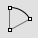##### Arc

Draw an arc with options for center, start, angle, and direction.##### Arc, Start, End, Direction

Arc by start, end, direction at start.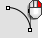##### Arc, Start, Direction, End

Arc by start, direction at start, end.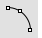##### Arc, Start, End, Point

Arc by start, end, point on arc.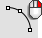##### Arc, Start, Point, End

Arc by start, point on arc, end.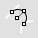##### Arc, Tangent

Arc tangent to curves.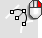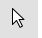##### Cancel

Cancel the current command and deselects objects.##### Cancel,All

Turns off Points, CurvatureGraph, EMap, Zebra, MoveUVN, CurvatureAnalysis, and deselects objects.##### Catenary

Creates a curve that a hanging chain or cable assumes under its own weight when supported only at its ends.##### Circle

Draw a circle from center and radius, diameter, points on the circumference, and circumference length.##### Circle, Diameter

Draws a circle from points on diameter.Draws a circle tangent to two curves and a radius.##### Circle, Vertical to CPlane: center, radius

Draws a circle from a center and radius vertical to construction plane.##### Conic, Tangent at start

Draw a conic curve tangent at the start.##### Conic, Tangent at start and end

Draw a conic curve tangent at the start and end.##### Curve

Draw a curve from control point locations.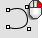##### CurveThroughPt

Fit a curve through point objects.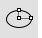##### Ellipse

Draw a closed elliptical curve from focus points, center and edges, bounding rectangle, and around a curve.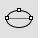##### Ellipse, Diameter

Draws a closed elliptical curve by diameter.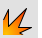##### Explode

Break objects down into components.##### ExtractSrf

Separates or duplicates polysurface, Extrusion, or SubD faces.##### HandleCurve

Draw chained Bézier curves with editing handles.##### Helix

Draw a helical curve with options for number of turns, pitch, vertical, reverse, and around a curve.##### Helix, Vertical

Draw a vertical helical curve.##### InterpCrv

Fit a curve through picked locations.

#####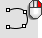HandleCurve

Draw chained Bézier curves with editing handles.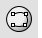##### InterpCrvOnSrf

Fit a curve through locations on a surface.##### Join

Connect curves, surface edges, or surfaces to form a single object.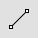##### Line

Draw a single line segment.##### Line, Normal

Draw a line segment normal to a surface.##### Line, Normal, BothSides

Draw a line segment normal to a surface from its midpoint.##### Line, Perpendicular, from curve

Draw a line segment perpendicular from a curve.##### Line, Perpendicular, to curve

Draw a line segment perpendicular to a curve.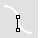##### Line, Tangent

Draw a line segment tangent from a curve.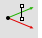##### Line, Vertical

Draw a line segment perpendicular to the construction plane.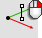##### Line, Vertical, BothSides

Draw a line segment perpendicular to the construction plane from its midpoint.##### Move

Move objects from one location to another.##### Parabola

Draw a parabolic curve from focus and vertex or endpoint.##### Parabola3Pt

Draw a parabolic curve through three picked points.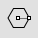##### Polygon

Draw a polygon with a specified number of sides with options for inscribed/circumscribed, by edge, star-shaped, around a curve, and vertical.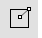##### Polygon, Square from center and corner

Draw a square polygon by center and corner.##### Polygon, Square, from edge

Draw a square polygon by edge.##### Polygon, Star

Draw a polygon star.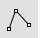##### Polyline

Draw a multi-segment polyline with options for line and arc segments, tracking line helpers, and close.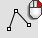##### Lines##### Rectangle

Draw a rectangular closed polyline from with options for starting at the center, three points, vertical, and rounded with arc or conic corners.##### Rectangle, 3Point

Draw a rectangle through three points.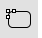##### Rectangle, Rounded

Draw a rectangle with rounded corners.##### Rectangle, Rounded, conic corners

Draw a rectangle with conic rounded corners.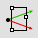##### Rectangle, Vertical

Draw a rectangle perpendicular to the construction plane.##### Sketch

Drag the mouse to draw a curve.##### Sketch, on surface

Drag the mouse to draw a curve on a selected surface.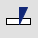##### Split

Divide objects using other objects as cutters.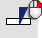##### Split,Isocurve

Divide objects using isocurves as cutters.##### Trim

Cut and delete selected portions of an object at the intersection with another object.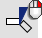##### Untrim

Remove trim curves from trimmed surfaces.

Rhinoceros 7 © 2010-2023 Robert McNeel & Associates. 03-May-2023